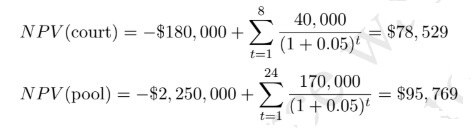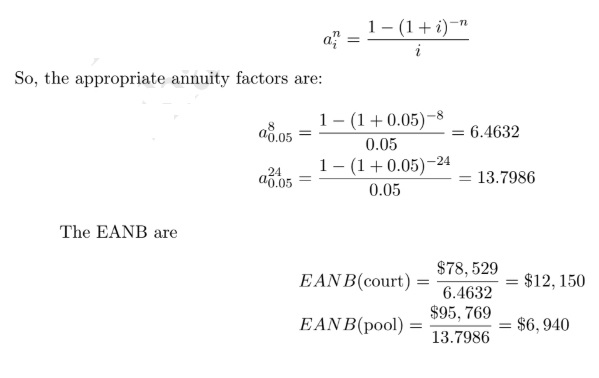# Econ 4831: Cost and Benefit Analysis

## Problem Set 2

Exercise 1: Discounting Benefits and Costs

A highway department is cosidering building a temporary bridge to cut travel time during the three years it will take to build a permanent bridge. The temporary bridge can be put up in a few weeks at a cost of \$740,000. At the end of three years, it would be removed and the steel would be sold for scrap. The real net costs of this would be \$81,000. Based on estimated time savings and wage rates, fuel savings, and reductions in risks of accidents, department analysts predict that the benefits in real dollars would be \$275,000 during the first year, \$295,000 during the second year,and \$315,000 during the third year. Department regulations require use of a real discount rate of 4 percent.

a) (5 pts) Calculate the present value of net benefits assuming that the benefits are realized at the end of each of the three years.

First, begin by calculating the PV of the costs. This includes the construction cost of the temporary bridge, which occurs at the beginning of year 1 and the net cost of decommissioning at the end of year 3:

P V (cost) = \$740, 000 + \$81, 000/(1 + 0.04)3
= \$740, 000 + \$72, 009 = \$812, 009

If the benefits accrue at the end of the year:

P V (benefits) = \$275, 000/(1 + 0.04) + \$295, 000/(1 + 0.04)2 + \$315, 000/(1 + 0.04)3

= \$264, 423 + \$272, 744 + \$280, 034

= \$817, 201

and the NPV is:

NPV = \$817, 201 \$812, 009 = \$5, 192

(b) (5 pts) Calculate the present value of net benefits assuming that the benefits are realized at the beginning of each of the three years.

If the benefits accrue at the beginning of the year:

P V (benefits) = \$275, 000 + \$295, 000/(1 + 0.04)1 + \$315, 000/(1 + 0.04)2

= \$275, 000 + \$283, 654 + \$291, 235

= \$849, 889

and the NPV is:

NPV = \$849, 889 \$812, 009 = \$37, 880

(c) (5 pts) Does the temporary bridge pass the net benefits test?

Notice that the sign of the NPV does not depend on when the benefits are assumed to occur. In both cases the NPV is positive, and they would pass the test.

Exercise 2: Projects with Di↵erent Time Length (20 points)

A town’s recreation department is trying to decide how to use a piece of land. One option is to put up basketball courts with an expected life of 8 years. Another is to install a swimming pool with an expected life of 24 years. The basketball court would cost \$180,000 to construct and yield net benefits of \$40,000 at the end of each of the 8 years. The swimming pool would cost \$2.25 million to construct and yield net benefits of \$170,000 at the end of each of the 24 years. Each project is assumed to have zero salvage value at the end of its life. Using a real discount rate of 5 percent, which project o↵ers larger net benefits? Approach the problem using the Roll-Over and the Equivalent Annual Net Benefit methods.

Consider first the NPV of each project separately:Notice that since the projects have di↵eretn length, it is not appropriate to choose based on NPV. First, use the Roll-Over method; that is, one could choose between one swimming pool and three successive basketball court projects.

NP V (3court) = \$78, 529 + \$78, 529/(1 + 0.05)8 + \$78, 529/(1 + 0.05)16

= \$78, 529 + \$53, 151 + \$35, 975

= \$167, 655

Thus, three successive basketball court projects o↵er a higher NPV of benefits than the swimming pool, and based on the Roll-Over one should build the basketball court. The other possible approach to the problem is using the Equivalent Annual Net Benefits. Remember that the annuity factor is equal toThe basketball court offers net benefits equivalent to an annuity paying \$12,304 each year over its life. The swimming pool o↵ers net benefits equivalent to an annuity paying \$6,942 each year over its life.

Exercise 3: Expected Benefits and Costs (20 points)

The initial cost of constructing a permanent dam (i.e., a dam that is expected to last forever) is

\$425 million. The annual net benefits will depend on the amount of rainfall: \$18 million in a ”dry” year, \$29 million in a ”wet” year, and \$52 million in a ”flood” year. Meteorological records indicate that over the last 100 years there have been 86 ”dry” years, 12 ”wet” years, and 2 ”flood” years. Assume the annual benefits, measured in real dollars, begin to accrue at the end of the first year. Using the meteorological records as a basis for prediction, what are the net benefits of the dam if the real discount rate is 5 percent.

The first step is to calculate the expected value of the annual net benefits:

(0.86) ⇥ \$18million + (0.12) ⇥ \$29million + (0.02) ⇥ \$52million = \$20million

The second step if to find the present value of the stream of annual net benefits. As the dam is assumed to be permanent, use the formula of the present value of a perpertuity:

\$20million

PV = = \$400million

0.05

The final step is to subtract the cost of construction from the present value of the annual benefit stream to obtain the overall present value of expected net benefits:

\$400million \$425million = \$25million

Thus, the dam do not pass the net benefits test.

Exercise 4: Internal Rate of Return (5 points)

Consider Exercise 3 above. Based on the Internal Rate of Return, should the dam be built? Explain.

The IRR is the discount rate at which the NPV is zero. In Exercise 3, make the PV of a perpetuity equal to its cost:

\$20 million

= \$425 million ) d = 0.047 d

Exercise 5: Option Price and Option Value (40 points)

Consider the project of constructing a dam. The only person a↵ected by this project is a farmer, with utility U(I) where \$I is his income. There are two possible contingencies: it rains a lot (wet) or it does not rain a lot (dry). With the dam, his income is \$160 if wet and \$100 if dry. Without the dam, his income is \$100 if Wet and \$80 if dry. The probability of raining a lot is 50%.

• (10 pts) What is the expected surplus of the farmer?

DAM NO DAM PROB

WET 160 100 0.5

DRY 100 80 0.5

The surplus if a wet contingency happens:

U(160 Sw) = U(100) ) Sw = 60

The surplus if a dry contingency happens:

U(100 Sd) = U(80) ) Sd = 20

Expected surplus is:

E(S) = 0.5 ⇥ 60 + 0.5 ⇥ 20 = 40

• (10 pts) Assume U(I) = ln I. What is his expected utility in case the dam is not constructed? What is his option price? Compare its option price to its expected surplus.

In case the dam is not constructed, farmer’s expected utility:

E[UNO] = 0.5 ⇥ U(100) + 0.5 ⇥ U(80)

= 0.5 ⇥ ln(100) + 0.5 ⇥ ln(80)

⇡ 0.5 ⇥ 4.6051 + 0.5 ⇥ 4.3820

= 4.493

Option price is the maximum an agent would accept to pay for the dam; that is, the farmer would accept to pay a value that makes him indi↵erent between the dam or not, E[UOP ] = E[UNO] where

E[UOP ] = 0.5 ⇥ U(160 OP )+ 0.5 ⇥ U(100 OP )

So, we have,

0.5 ⇥ ln(160 OP )+ 0.5 ⇥ ln(100 OP ) = 0.5 ⇥ ln(100) + 0.5 ⇥ ln(80)

ln(160 OP ) + ln(100 OP ) = ln(100) + ln(80)

ln[(160 OP ) ⇥ (100 OP )] = ln(100 ⇥ 80)

(160 OP ) ⇥ (100 OP ) = 100 ⇥ 80

16000 160OP 100OP + OP 2 = 8000

OP 2 260OP + 8000 = 0

To apply Bhaskara formula, let = b2 4ac. So,

= ( 260)2 +4 ⇥ 8000 = 67600 32000 = 35600

Then, we find that:

p

OP =

) OP1 = 35.66 or OP2 = 224.35

The solution is OP = 35.66. Notice that OP = 224.35 would lead the farmer to have negative income. Then we have that,

OP = 35.66 < 40 = E(S)

Farmer’s option price is lower than the expected surplus of the project. This is a consequence of the increase in risk (in terms of a higher variability in the income stream).

• (10 pts) Assume U(I) = I. What is his expected utility in case the Dam is not constructed? What is his option price? Compare its option price to its expected surplus.

In case the dam is not constructed, farmer’s expected utility:

E[UNO] = 0.5 ⇥ U(100) + 0.5 ⇥ U(80)

= 0.5 ⇥ 100 + 0.5 ⇥ 80

= 50 + 40

= 90

Also, consider the utility:

E[UOP ] = 0.5 ⇥ U(160 OP )+ 0.5 ⇥ U(100 OP )

= 0.5 ⇥ (160 OP )+ 0.5 ⇥ (100 OP )

= 80 OP/2 + 50 OP/2

= 130 OP

To compute the option price, make E[UOP ] = E[UNO]:

130 OP = 90

OP = 40

Then, we have that OP = 40 = E(S). Farmer’s option price is the same as the expected surplus of the project. This happens because the farmer does not value the increase in risk (in terms of a higher variability in the income stream). The farmer does not lose utility from increased consump- tion variability and only cares about expected income.

(d) (10 pts) Calculate the option value for each case above. Compare them. How would you explain their di↵erence?

For the risk averse famer, option value is:

OV = 35.66 40 = 4.44

and for the risk neutral farmer:

OV = 40 40 = 0

The di↵erence is due to the e↵ects of risk for each type of farmer. While the risk averse loses utility from the increase in risk, the risk neutral is indi↵erent to it.

Extra Points Exercise: Sensitivity Analysis (30 points)

Reproduce Figure 7.3 and Figure 7.5 in the book. Together with the figures, explain the methodol- ogy to compute them; i.e., the idea behind the method and how you did it. The explanation should have about ten to twelve lines.